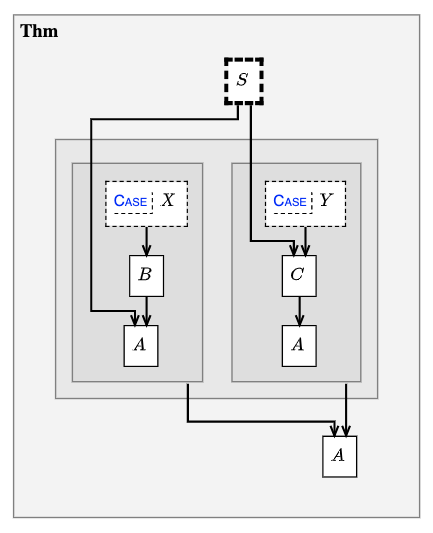# Contradictions and Cases#

Proof by contradiction and proof by cases are two common forms for mathematical proofs. Recalling the general node format (Listing 7), both of these proof structures are encoded using node `MODIFIERS`.

## Proof by contradiction#

Proofs by contradiction use the `contra` modifier.

To encode a proof by contradiction:

• Deduce a `flse` node from a set of nodes (often just two) that are mutually contradictory.

• Link the `flse` node to a `supp` node using the `contra` modifier.

Example:

Listing 14 A proof by contradiction#
```deduc Thm {
supp S {
sy="\$S\$"
}
asrt A {
sy="\$A\$"
}
meson="Suppose S. Then A."
}

deduc Pf of Thm.A {

supp T {
en="Suppose not \$A\$."
}

asrt B {
sy="\$B\$"
}

asrt C {
sy="\$C\$"
}

asrt D {
sy="\$D\$"
}

flse F contra T {}

meson = "
Suppose T. Then B and C. From C get D, using Thm.S, hence F, by B.
Therefore Thm.A.
"
}
```

## Proof by cases#

Proofs by cases use the `versus` modifier.

To encode a proof by cases:

• Use a set of `subdeduc` nodes, one for each case.

• Start each `subdeduc` with a `supp` node, indicating the assumption that defines the case.

• On one of these `supp` nodes, use the `versus` modifier to link it with the alternative cases.

• Deduce the common conclusion, citing each of the `subdeduc` nodes as reason.

Example:

Listing 15 A proof by cases#
```deduc Thm {
supp S {
sy="\$S\$"
}
asrt A {
sy="\$A\$"
}
meson="Suppose S. Then A."
}

deduc Pf of Thm.A {

subdeduc caseX {
supp X {
sy="\$X\$"
}
asrt B {
sy="\$B\$"
}
asrt A {
sy="\$A\$"
}
meson = "Suppose X. Then B, hence A, by Thm.S."
}

subdeduc caseY {
supp Y versus caseX.X {
sy="\$Y\$"
}
asrt C {
sy="\$C\$"
}
asrt A {
sy="\$A\$"
}
meson = "Suppose Y. Then C by Thm.S, hence A."
}

meson = "Thm.A by caseX and caseY."

}
```Fig. 15 A proof by cases#

## Using auxiliary deducs#

It is often a good idea to write skeletal proofs in each subdeduc node, while writing a separate deduc to fill in the argument, so that the subdeduc can be expanded and collapsed.

An example of this technique can be seen in a proof by cases, each part of which is a proof by contradiction, in the proof of FLT for regular primes, from Hilbert’s Zahlbericht.